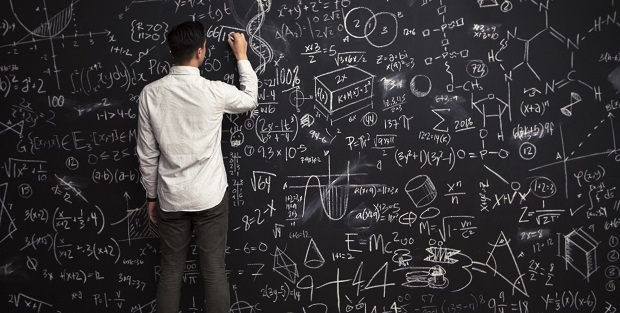# GCSE Maths – Standard FormNumbers that are very large or very small can be awkward to write down, so there is a standardised form for writing all numbers;

A × 10n, where 1 ≤ A < 10 and n is an integer.

Examples

36520 = 3.652 x 104, 0.0247 = 2.47 x 10-2, 473= 4.73 x 102, 9.45 x 10-3 = 0.00945

Multiplying two standard form numbers is quite straightforward; just multiply the two As and add the two ns

Examples

36520 x 0.0247 = (3.652 x 2.47) x 10(4-2) = 9.02044 x 102

36520 x 473 = (3.652 x 4.73) x 10(4+2) = 17.27396 x 106 = 1.727396 x 107

Adding or subtracting two standard form numbers requires a little bit more care as the two numbers must first be expressed in terms of the same power of 10

Examples

3.652 x 104 + 4.73 x 102 = 365.2 x 102 + 4.73 x 102 = 369.93 x 102 = 3.6993 x 104

2.47 x 10-2 + 9.45 x 10-3 = 24.7 x 10-3 + 9.45 x 10-3 = 34.15 x 10-3 = 3.415 x 10-2

### Work Towards That 'A' Grade

Achieve the results you want with-written revision resources when you register with iRevise.
Its Free!